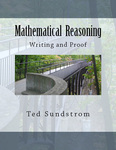## Open Textbooks

#### Title

Mathematical Reasoning: Writing and Proof

#### Authors

Mathematical Reasoning: Writing and Proof Version 2.1 is also available: https://scholarworks.gvsu.edu/books/9/

#### Files

Note to Students (59 KB)

Preface (103 KB)

Introduction to Writing Proofs in Mathematics (327 KB)

Logical Reasoning (462 KB)

Constructing and Writing Proofs in Mathematics (824 KB)

Mathematical Induction (460 KB)

Set Theory (642 KB)

Functions (834 KB)

Equivalence Relations (525 KB)

Topics in Number Theory (398 KB)

Finite and Inﬁnite Sets (424 KB)

Appendix A: Guidelines for Writing Mathematical Proofs (87 KB)

Appendix B: Answers for the Progress Checks (401 KB)

Appendix C: Answers and Hints for Selected Exercises (279 KB)

Appendix D: List of Symbols (69 KB)

Index (89 KB)

#### Description

Mathematical Reasoning: Writing and Proof is designed to be a text for the ﬁrst course in the college mathematics curriculum that introduces students to the processes of constructing and writing proofs and focuses on the formal development of mathematics. The primary goals of the text are to help students:

• Develop logical thinking skills and to develop the ability to think more abstractly in a proof oriented setting.

• Develop the ability to construct and write mathematical proofs using standard methods of mathematical proof including direct proofs, proof by contradiction, mathematical induction, case analysis, and counterexamples.

• Develop the ability to read and understand written mathematical proofs.

• Develop talents for creative thinking and problem solving.

• Improve their quality of communication in mathematics. This includes improving writing techniques, reading comprehension, and oral communication in mathematics.

• Better understand the nature of mathematics and its language.

Another important goal of this text is to provide students with material that will be needed for their further study of mathematics.

This type of course has now become a standard part of the mathematics major at many colleges and universities. It is often referred to as a “transition course” from the calculus sequence to the upper-level courses in the major. The transition is from the problem-solving orientation of calculus to the more abstract and theoretical upper-level courses. This is needed today because many students complete their study of calculus without seeing a formal proof or having constructed a proof of their own. This is in contrast to many upper-level mathematics courses, where the emphasis is on the formal development of abstract mathematical ideas, and the expectations are that students will be able to read and understand proofs and be able to construct and write coherent, understandable mathematical proofs. Students should be able to use this text with a background of one semester of calculus.

7-2013

#### Keywords

proofs course, transition course, mathematical writing, proof writing

Screencasts designed for use with the book can be found at http://www.youtube.com/playlist?list=PL2419488168AE7001.

This book has been reviewed by the American Institute of Mathematics Open Textbook Initiative. http://www.aimath.org/textbooks/